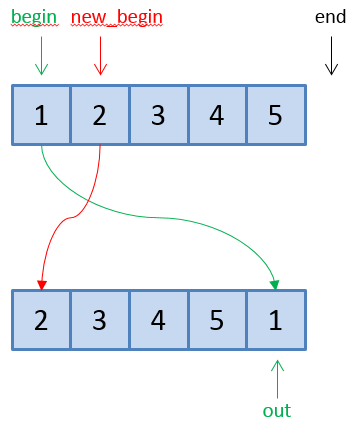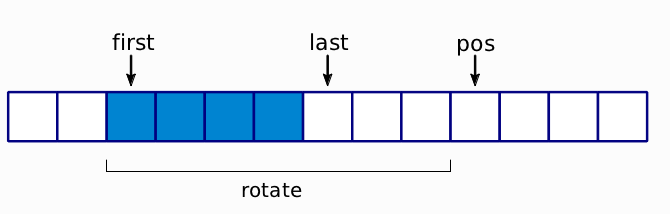# STL 中容器重新排列的方法

STL 库可以对容器做很多操作， 其中一个就是可以对容器中的元素进行重新排序。或者换句话说，可以对容器进行组合排列(premutation)。

• 字典序 排列
• 循环 排列
• 随机 排列
• 逆序
• 检查排列
• 其他排列方式

## 字典序 排列

{1, 2, 3, 4, 5}
{1, 2, 3, 5, 4}
{1, 2, 4, 3, 5}
{1, 3, 4, 5, 3}
...


vector<int> v = {1, 2, 3, 4, 5 };

std::next_permutation(v.begin(), v.end()); // v 现在包含 {1, 2, 3, 5, 4}


std::next_premutation 返回一个 bool 值， 如果得到的排列是字典序大于原排列 (任意一个大于排列) 就返回 true, 否则返回 false (唯一的例子是下一个迭代返回到了之前第一个（最小的）排列方式)。

vector<int> v = {1, 2, 3, 5, 4};

std::prev_permutation(v.begin(), v.end()); // v now contains {1, 2, 3, 4, 5 }


std::next_premutationstd::prev_premutation 直接作用在输入参数指定的范围内， 这可以方便的多次调用他们：

std::vector<int> numbers = {1, 2, 3, 4};
while (std::next_permutation(begin(numbers), end(numbers)))
{
for (int n : numbers) std::cout << n << ' ';
std::cout << '\n';
}


1 2 4 3
1 3 2 4
1 3 4 2
1 4 2 3
1 4 3 2
2 1 3 4
2 1 4 3
2 3 1 4
2 3 4 1
2 4 1 3
2 4 3 1
3 1 2 4
3 1 4 2
3 2 1 4
3 2 4 1
3 4 1 2
3 4 2 1
4 1 2 3
4 1 3 2
4 2 1 3
4 2 3 1
4 3 1 2
4 3 2 1


## 循环 排列

{1, 2, 3, 4, 5}
{5, 1, 2, 3, 4}
{4, 5, 1, 2, 3}
{3, 4, 5, 1, 2}
{2, 3, 4, 5, 1}


### 基本使用方法

std::rotate 使用 3 个 迭代器：

• 一个指向排列范围开始
• 一个指向你想把它排在最前面的元素
• 一个指向排列范围的末尾

template<typename ForwardIterator>
ForwardIterator rotate(ForwardIterator begin, ForwardIterator new_begin, ForwardIterator end);template<typename ForwardIterator>
void rotate(ForwardIterator begin, ForwardIterator new_begin, ForwardIterator end);


std::rotate 直接作用（修改）传入的排列范围内的元素。如果你想保持原范围元素不变，可以使用 std::rotate_copy 将输出保存到另外一个容器中。

### 一个 std::rotate 的有趣用法

std::rotate 可以用来创建新的算法， Sean Parent 在 GoingNative 2013 上做过的 C++ Seasoning 中提到过这个事情。让我们来看一下 Sean 当时的例子， 这个例子展示了 STL 的能力。std::rotate(first, last, pos);std::rotate(pos, first, last);


if (pos < first) std::rotate(pos, first, last);
if (last < pos) std::rotate(first, last, pos);


• 如果 pos < first， 滑动后的元素位于 pos 和 排列后新的第一个元素之间 （不是 滑动区间）， 这是 std::rotate（pos, first, last） 的返回值。
• 如果 last < pos， 滑动后的元素位于排列后第一个元素和 pos 之间

template <typename ForwardIterator>
std::pair<ForwardIterator, ForwardIterator> slide(ForwardIterator first, ForwardIterator last, ForwardIterator pos)
{
if (pos < first) return { pos, std::rotate(pos, first, last) };
if (last < pos) return { std::rotate(first, last, pos), pos };
return { first, last };
}


## 随机 排列

std::shuffle 可以用来做这个：

#include <random>
#include <algorithm>
#include <vector>

std::vector<int> numbers = {1, 2, 3, 4, 5, 6, 7, 8, 9, 10};

std::random_device randomDevice;
std::mt19937 generator(randomDevice());

std::shuffle(begin(numbers), end(numbers), generator);

for (int n : numbers) std::cout << n << ' ';


8 10 5 1 7 2 3 6 4 9


## 逆序 排列

std::vector<int> numbers = {1, 2, 3, 4, 5, 6, 7, 8, 9, 10};

std::reverse(begin(numbers), end(numbers));


10 9 8 7 6 5 4 3 2 1


## 排列的检查

std::vector<int> v1 = {1, 2, 3, 4, 5};
std::vector<int> v2 = {4, 2, 3, 1, 5};
std::vector<int> v3 = {2, 3, 4, 5, 6};


std::is_permutation(v1.begin(), v1.end(),
v2.begin(), v2.end());


std::is_permutation(v1.begin(), v1.end(),
v3.begin(), v3.end());


std::is_permutation(v1.begin(), v1.end(),
v2.begin());


std::is_permutationoperator== 进行元素比较， 并且可以接受自定义的比较函数。

### std::is_permutation 的算法复杂度

std::is_permutation 具有至多 ”O(n)“ 的复杂度。

### std::is_permutation 的一个使用例子

std::vector<int> expected = {1, 2, 3, 4, 5};

std::vector<int> results = f();

EXPECT_TRUE(std::is_permutation(begin(expected), end(expected),
begin(results), end(results)));